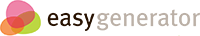# Two digit by Two digit multiplication

## Two digit by two digit multiplication

### True/False assessment

• When multiplying 2 digit numbers, you start by multiplying from the 10's column first.
• You always need to put a "place holder" after you are done multiplying from the ones column.